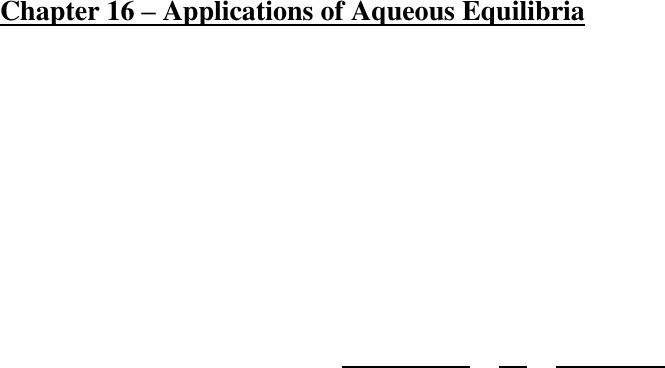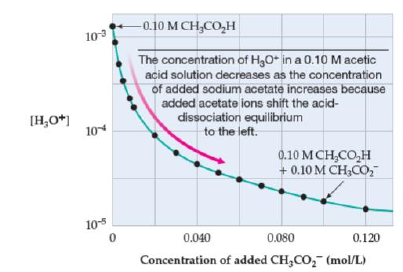# CHM135H1 Chapter Notes - Chapter 16: Weak Base, Acid Strength, Chemical Equation

199 views10 pages
School
Department
CourseCHM135 CHEMISTRY TEXTBOOK CH. 16 NOTES/SUMMARY
Chapter 16 Applications of Aqueous Equilibria
Chapter 16.1: Neutralization Reactions
- Looking at four types of neutralization reactions
1. Strong acid strong base
2. Weak acid strong base
3. Strong acid weak base
4. Weak acid weak base
- Strong Acid Strong Base
o Since the neutralization reaction of any strong acid with a strong base is the reverse of the
dissociation of water, its equilibrium constant, Kn (“n” for neutralization), is the
reciprocal of the ion product constant for water, Kn = 1/Kw
o H3O+(aq) + OH-(aq) → 2 H2O(l)

 
o The value of Kn for a strong acid strong base reaction is a very large number, which
means that the neutralization reaction proceeds essentially 100% to completion
o After neutralization of equal molar amounts of acid and base, the solution contains a salt
derived from a strong base and a strong acid neither the cation nor the anion of the salt
has acidic or basic properties, the pH is 7
- Weak Acid Strong Base
o Since a weak acid HA is largely undissociated, the net ionic equation for the
neutralization reaction of a weak acid with a strong base involves proton transfer from
HA to strong base OH-:
HA(aq) + OH-(aq) → H2O(l) + A-(aq)
o Net reaction equals the product of the equilibrium constants for the reactions added
o As a general rule, the neutralization of any weak acid with a strong base will go 100% to
completion because OH- has a great affinity for protons
o pH is greater than 7
- Strong Acid Weak Base
o A strong acid HA is completely dissociated into H3O+ and A- ions, and its neutralization
reaction with a weak base therefore involves proton transfer from H3O+ to the weak base
B:
H3O+(aq) + B(aq) → H2O(l) + BH+(aq)
o The equilibrium constant for the neutralization reaction is given by multiplying
equilibrium constants for reactions that add to give the net ionic equation
o The neutralization of any weak base with a strong acid generally goes 100% to
completion because H3O+ is a powerful proton donor
o pH is less than 7
- Weak Acid Weak Base
o Both a weak acid HA and a weak base B are largely undissociated, and the neutralization
reaction between them therefore involves proton transfer from the weak acid to the weak
base
o Equilibrium constant Kn can be obtained by multiplying equilibrium constants for the
o The value of Kn is smaller than it was for the previous cases indicating that the
neutralization doesn’t proceed as far toward completion
Unlock document

This preview shows pages 1-3 of the document.
Unlock all 10 pages and 3 million more documents.o In general, weak acid weak base neutralizations have less tendency to proceed
completion than neutralizations involving strong acids or strong bases
Chapter 16.2: The Common Ion Effect
- Solution of weak acid and its conjugate base is an important acid base mixture because such
mixtures regulate pH in biological systems
- To illustrate pH calculations for weak acid conjugate base mixtures:
1. Classify species as acidic, basic, or inert
2. Write proton transfer reactions and identify principal reaction
3. Set up an equilibrium table for the principal reaction
4. Substitute the equilibrium concentrations into the equilibrium equation for the
principal reaction and solve for x
5. Calculate the concentrations of species involved in the equilibrium reactions
6. Calculate the pH
(Textbook, Page 662)
- The common ion effect is another example of Le Chatelier’s principle in which stress on
equilibrium of raising one of the product concentrations is relieved by shifting equilibrium to
reactant side
Chapter 16.3: Buffer Solutions
- Solutions containing a weak acid and its conjugate base are buffer solutions because they resist
drastic changes in pH
- Buffer solution Weak acid (HA) + Conjugate base (A-)
o CH3CO2H + CH3CO2-
o HF + F-
o NH4 + NH3
o H2PO4- + HPO42-
- If small amount of OH- is added to a buffer solution, pH increases, but not by much, because acid
component of buffer solution neutralizes added OH-
HA(aq) + OH-(aq) H2O(l) + A-(aq)
- If small amount of H3O+ is added to buffer solution, but pH barely decreases because base
component of buffer solution neutralizes H3O+
A-(aq) + H3O+(aq) H2O(l) + HA(aq)
- Buffer solutions very important in biological systems blood is a buffer solution that can reduce
pH changes when acids and bases are produced in biological reactions; cardiac arrest can add acid
to blood due to buildup of carbon dioxide in blood; hyperventilation increases amount of carbon
dioxide removed from body and raises blook pH
- pH of human blood is controlled in pH range of 7.35 to 7.45 by conjugate acid base pairs,
primarily H2CO3 and its conjugate base HCO3-
Unlock document

This preview shows pages 1-3 of the document.
Unlock all 10 pages and 3 million more documents.- oxygen carrying ability of hemoglobin in blood depends on control of pH to within 0.1 pH unit
- For commonly used buffer solutions, Ka is small and initial concentrations relatively large x is
generally negligible compared with initial concentrations, so initial concentrations can be used in
calculations
- (Textbook, Page 667)
- When OH- or H3O+ is added to buffer solution, ratio of weak acid to conjugate base change; if
amount added is small compared to amount of weak acid and base in buffer, then ratio and pH
will only change slightly
- Buffer capacity is a measure of the amount of acid or base that the solution can absorb without a
significant change in pH; also a measure of how little pH changes with addition of a given
amount of acid or base
- Buffer capacity dependent on amount of moles of weak acid and conjugate base present for
equal volumes of solution, more concentrated solution, the greater the buffer capacity; for
solutions having same concentrations, greater the volume, the greater the buffer capacity
Chapter 16.4: The Henderson Hasselbalch Equation
- [H3O+] in a buffer solution depends on dissociation constant of weak acid and on concentration
ratio [weak acid]/[conjugate base]:





Because pKa = - log Ka and



- Henderson Hasselbalch equation:


- Equation states that pH of buffer solution has value close to pKa of weak acid; when
[base]/[acid]=1, then the log [base]/[acid]=0 and pH equals pKa
- Importance of Henderson Hasselbach equation in biochemistry is that it tells us how pH affects
% dissociation of a weak acid
- Equation also tells us how to prepare buffer solution with given pH general idea is to select
weak acid whose pKa is close to desired pH and then adjust ratio of [base] to [acid] to value
specified by Henderson Hasselbalch equation
- Since buffer solutions are widely used in laboratory and in medicine, prepackaged buffers having
variety of precisely known pH values are commercially available manufacturers prepare
buffers by choosing buffer system having an appropriate pKa value and adjusting amounts of
ingredients to achieve proper value
Unlock document

This preview shows pages 1-3 of the document.
Unlock all 10 pages and 3 million more documents.

# Get access

\$10 USD/m
Billed \$120 USD annually
Homework Help
Class Notes
Textbook Notes